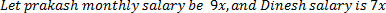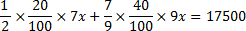## Percentage For SBI PO : Set – 02

1) 15 percent of the voters in an election did not cast their votes. In this election there are only two candidates. The winner by obtaining 48% of the total votes and defeated his rival by 22000 votes. The total number of votes in the election?

a) 100000

b) 175000

c) 200000

d) 125000

e) None of these

c)

15% didn’t cast their vote. 48% of total votes get by the winning candidates, so remaining 37% will be scored by his rival. So,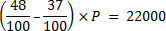P = 200000

2) In a competition the percentage of students qualified to the number of students Participated from school ‘X’ is 60%. In school ‘Y’ the number of students Participated is 30% more than the students Participated from school ‘X’ and the number of students qualified from school ‘Y’ is 60% more than the students qualified from school ‘X’. What is the percentage of students qualified to the number of students Participated from school ‘Y’?

a) 70%

b) 75%

c) 72%

d) 73 11/3%

e) None of these

c)

Let students Participated from school X = 100

Qualified students from school X = 60

Now, student Participated from school Y = 130

And Qualified student from school Y = 60 + 36 = 96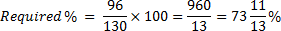3) Ravi attend science test consist of 85 questions from three sections- i.e. A, B and C. 10 questions from section A, 30 questions from section B and 45 question from section C. Although, she answered 70% of section A, 50% of section B and 60% of section C correctly. She did not pass the test because she got less than 60% of the total marks. How many more questions she would have to answer correctly to earn 60% of the marks which is passing grade?

a) 4

b) 2

c) 5

d) 6

e) 8

b)

Number of Questions attempt correctly=70% of 10+50% of 30+60% of 45

=7+15+27=49

required mark is=51-49=2 marks.

4) Mrs. Sharma invests 15% of her monthly salary, i.e., Rs. 4428 in Mutual Funds. Later she invests 18% of her monthly salary on Pension Policies also she invests another 9% of her salary on Insurance Policies. What is the total monthly amount invested by Mrs. Sharma?

a) Rs. 113356.8

b) Rs. 12398.4

c) Rs. 56678.4

d) Rs.13454.7

e) Can’t determined

b)

15%=4428

1%=4428/15

(15+18+9)=42%

4428/15×42=Rs.12398.4

5) In an examination Parvin scored 25 marks less than Ahmed. Ahmed scored 45 more marks than Rithik. Tamil scored 75 marks which is 10 more than Rithik. Senthil’s score is 80 less than maximum marks of the test. What approximate percentage of marks did Senthil score in the examination if he gets 55 marks more than Rithik?

a) 90

b) 70

c) 80

d) 60

e) 85

d)

Marks of Tamil = 75

Marks of Rithik = 65

Marks of Ahmed = 110

Marks of Parvin = 85

Marks of senthil = 120

Maximum marks = 200

Required percentage =120/200×100=60%

6) A school has raised 75% of the amount it needs for a new building by receiving an average donation of Rs. 1200 from the parents of the students. The people already solicited represents the parents of 60% of the students. If the School is to raise exactly the amount needed for the new building, what should be the average donation from the remaining students to be solicited?

a) Rs.800

b) Rs.900

c) Rs.850

d) Rs.600

e) Rs.720

d)

Let the number of parents be x who has been asked for the donations.

People already solicited = 60% of x = 0.6x

Remaining people = 40% of x = 0.4x

Amount collected from the parents solicited= 1200 ×0.6x = 720x

720x = 75%; Remaining amount = 25% = 240x

Thus, Average donations from remaining parents = 240X/0.4X=600

7) In an examination, 1100 boys and 900 girls appeared. 50% of the boys and 40% of the girls passed the examination. What is the percentage of candidates failed?

a) 45

b) 45.5

c) 50

d) 54.5

e) None of these

d)

Failed candidates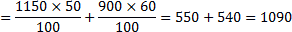Required percentage =(1090/2000)*100=54.5

8) A dealer offered a machine for sale 27500, but even if had charged 10% less, he would have made a profit of 10%. The actual cost of the machine is

a) 22500

b) 24250

c) 22275

d) 22000

e) None of these

a)

10% of 27500 = Rs.2750

S.P = 27500-2750

Rs.24750

C.P=Rs.22500

9) In a university election, in which there were only  two candidates; 10% of the voting list did not vote and 60 votes cast were declared invalid. The successful candidate secured 47% of the total number on voting list and won by a margin of 308 votes. The number of voters on the voting list was

a) 6,000

b) 6200

c) 6,500

d) 7,000

e) None of these

b)

W + L = 0.9V – 60  …(i)

W – L  = 308  …(ii)

Adding equations (i) and (ii), we get  2 W = 0.9 V + 248

2 x 0.47 V = 0.9V + 248

V = 6200

10) The ratio of the monthly salary of Dinesh to that Prakesh is 7:9. Dinesh and Prakesh both save 20% and 40% of their respective monthly salary respectively. Dinesh invests ½ of his savings in PPF and Prakesh invests 7/9 of his savings in PPF. If Dinesh and prakesh together saved 17500 in PPF, what is Prakesh  monthly salary?

a) 46000

b) 35000

c) 45000

d) 55000

e) 45700